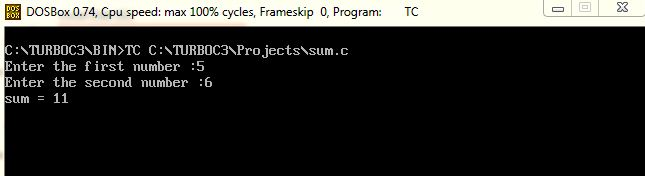UncleCoder.com

UncleCoder.com

Free programming examples and instructions

# Sample C Program

## Sample C Program for how to find sum of two integer numbers

by Athil

Posted on 27 Jun 2018 Category: C Views: 300

Sample C Program to find Sum of any two numbers

In this section, we will write a sample or basic C program to find the sum of two numbers. The source code and ouput is shown below.

Here the user reads two numbers using 'scanf()' and print the number using 'printf()' function.

``````#include<stdio.h>

int main() {
int a, b, sum;

printf("\nEnter the first number: ");
scanf("%d ", &a);
printf("\nEnter the second number: ");
scanf("%d ", &b);

sum = a + b;

printf("sum= %d", sum);

return(0);
}``````

OUTPUTRelated Articles

C program to find sum of first 10 integers using for loop

C program to swap two numbers without using third variable

C program to find largest among two numbers

C Program to find GCD of two numbers

C program to find Reverse of a Number

C program to find the factors of a number

C program to convert decimal to binary

### Latest posts in C

##### C program to find largest among two numbers• View all

• View all

• View all

• View all

• View all